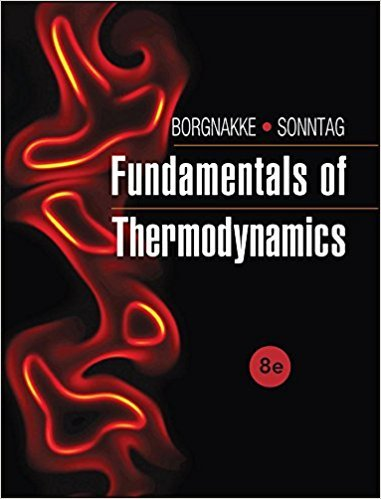# A gasoline engine has a piston/cylinder with 0.1 kg air at## Problem 156HP Chapter 3

Fundamentals of Thermodynamcs | 8th Edition

• 2901 Step-by-step solutions solved by professors and subject experts
• Get 24/7 help from StudySoup virtual teaching assistantsFundamentals of Thermodynamcs | 8th Edition

4 5 0 396 Reviews
26
1
Problem 156HP

A gasoline engine has a piston/cylinder with 0.1 kg air at 4 MPa, 1527°C after combustion, and this is expanded in a polytropic process with n = 1.5 to a volume 10 times larger. Find the expansion work and heat transfer using the heat capacity value in Table A.5.

TABLE A.5

Properties of Various Ideal Gases at 25°C, 100 kPa*(SI Units)

 Gas ChemicalFormula Molecular Mass(kg/kmol) R(kJ/kg-K) ρ (kg/m3) Cp0(kJ/kg-K) Cv0(kJ/kg-K)Steam H2O 18.015 0.4615 0.0231 1.872 1.410 1.327 Acetylene C2H2 26.038 0.3193 1.05 1.699 1.380 1.231 Air — 28.97 0.287 1.169 1.004 0.717 1.400 Ammonia NH3 17.031 0.4882 0.694 2.130 1.642 1.297 Argon Ar 39.948 0.2081 1.613 0.520 0.312 1.667 Butane C4H10 58.124 0.1430 2.407 1.716 1.573 1.091 Carton dioxide CO2 44.01 0.1889 1.775 0.842 0.653 1.289 Carton monoxide CO 28.01 0.2968 1.13 1.041 0.744 1.399 Ethane C2H6 30.07 0.2765 1.222 1.766
Step-by-Step Solution:

Solution 156HP

Step 1 of 3</p>

We are required to calculate the expansion work and heat transfer during the given process.

Step 2 of 3</p>

The mass of air in the cylinder iskg

The given process is polytropic and the value ofis 1.5.

The initial specific volume isThe final specific volume isThe initial pressure isMPakPa

Let the final pressure be.

In a polytropic process,constant

Thus,kPa

The work done in a polytropic process is,, whereandare initial and final temperatures.C = 1800 K

From the ideal gas equation,,andTherefore,K

Substituteandvalues inJ [R for air is (8.314/ air molar mass= 28.97 g/mol)]kJ

Therefore, the work done is 70.6 kJ.

Step 3 of 3

##### ISBN: 9781118131992

Unlock Textbook Solution

A gasoline engine has a piston/cylinder with 0.1 kg air at

×
Get Full Access to Fundamentals Of Thermodynamcs - 8th Edition - Chapter 3 - Problem 156hp

Get Full Access to Fundamentals Of Thermodynamcs - 8th Edition - Chapter 3 - Problem 156hp

I don't want to reset my password

Need help? Contact support

Need an Account? Is not associated with an account
We're here to help